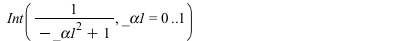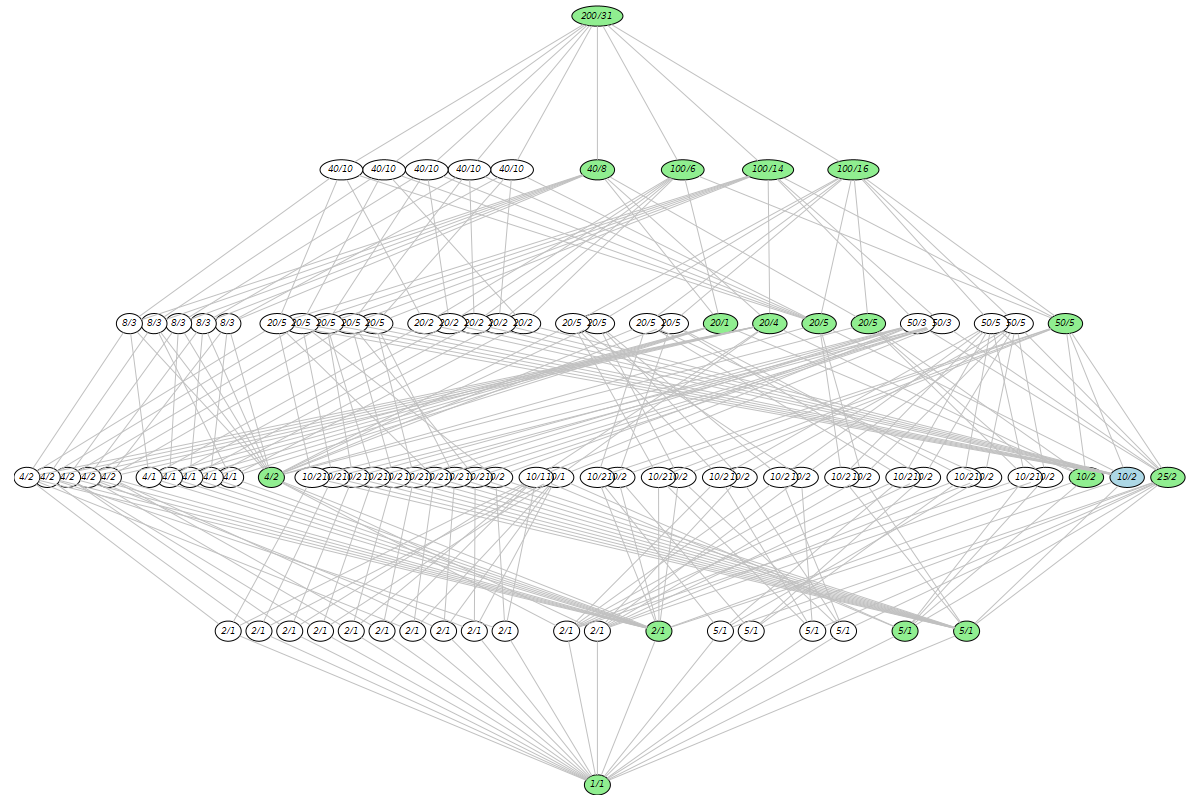## 11977 Reputation

7 years, 254 days

## Digits...

The denominator has many very small values ==> large roundoff errors.
Increasing Digits (e.g. Digits:=15) solves the problem.

## evalf...

If the matrix A has both exact and float entries, then it is obvious that some computations (e.g. the value of a discontinuous function) may return "unexpected" results.
Rank is such a discontinuous function. You have to accept this fact.
E.g. if A has two columns such that the first one is symbolic with at least an irrational entry,
and the second column is nonzero with float entries, the rank(A) is always 2.

 > restart;
 > v:=:
 > A:=;(1)
 > LinearAlgebra:-Rank(A);        # exact(2)
 > LinearAlgebra:-Rank(evalf(A)); # "approx" but probably "expected"(3)

## Just replace in param1,...,param4: ...

Just replace in param1,...,param4:  alpha*gamma=0.004;   with   gamma=0.004/alpha;
(or use algsubs).

- don't load packages which are not needed
- gamma is a Maple constant, don't use it as a variable
- linalg is deprecated; use LinearAlgebra instead.

## Int...

```convert(EllipticK(x), Int):
eval(%,x=1); value(%);```infinity

## size...

```with(GroupTheory):
g:=DrawSubgroupLattice(SmallGroup(200, 31), labels = ids, output=graph):
GraphTheory:-DrawGraph(g, size=[1200,800]);
```## prettyprint...

Execute

`pp:=interface(prettyprint=1);`

before Generators(...), then copy.
To restore the old settings:

```interface(prettyprint=pp):
```

## There are only a f...

There are only a few automatic simplifications. c*infinity  and  c+infinity  are not automatically simplified even when c is constant (containing symbolic objects).

 > Pi*infinity, Pi+infinity;(1)
 > (abs(x)+1)*infinity;(2)
 > simplify(%);